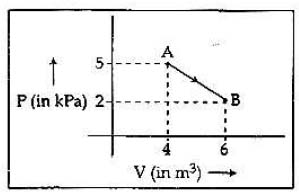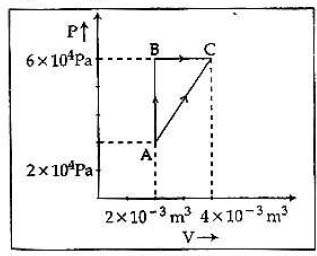## Filters

Sort by :
Clear All
Q
Medical
288 Views   |

One mole of an ideal diatomic gas undergoes a transition from A to B along a path AB as shown in the figure,The change in internal energy of the gas during the transition is:

• Option 1)

20 J

• Option 2)

-12 kJ

• Option 3)

20 kj

• Option 4)

-20 kJ

As we learnt in  Total internal energy -   - wherein Change in internal energy (Always) is degree of freedom   or     Option 1) 20 J Incorrect Option 2) -12 kJ Incorrect Option 3) 20 kj Correct Option 4) -20 kJ Incorrect
Medical
363 Views   |

Figure below shows two paths that may be taken by a gas to go from a state A to a state C.In process AB, 400 J of heat is added to the system and in process BC, 100 J of heat is added to the system. The heat absorbed by the system in the process AC will be:

• Option 1)

460 J

• Option 2)

300 J

• Option 3)

380 J

• Option 4)

500 J

As we learnt in  Work by area - If we plot a process on PV diagram.Then area under the curve will give work done. - wherein   , isochoric process  isobaric process                                        Correct option is 1.   Option 1) 460 J Correct Option 2) 300 J Incorrect Option 3) 380 J Incorrect Option 4) 500 J Incorrect
Medical
131 Views   |

A Carnot engine, having an efficiency ofas heat engine, is used as a refrigerator. If the work done on the system is 10 J, the amount of energy absorbed from the reservoir at lower temperature is:

• Option 1)

90 J

• Option 2)

1 J

• Option 3)

100 J

• Option 4)

99 J

As we learnt in  Coefficient of performance (beta) -   - wherein For a perfect refrigerator     AND     Efficiency of a carnot cycle -   are in kelvin   - wherein Source temperature Sink Temperature   Correct option is 1. Option 1) 90 J This option is correct Option 2) 1 J This option is incorrect Option 3) 100 J This option is incorrect Option 4) 99 J This option is incorrect
Exams
Articles
Questions